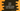# Java program to print a square using any character### Java program to print a square using a character :

This tutorial is on how to print a square like below using any character in Java :

``````& & & & &
&             &
&             &
&             &
& & & & & ``````

We will create the program to print the square by using any character like ’*’,’%’,’\$’ etc. Not only by ’&‘. Following algorithm we are going to use with this program :

### Algorithm we are using :

1. Take the size of the square from the user.
2. Take the character user wants to print the square.
3. Run one loop same as the size of the square.
4. Run one inner loop same as the outer loop , i.e. as the size of the square.
5. Outer loop indicates the row number and inner loop is used to print character for each row.
6. For the first and last run of the outer loop, print all characters in the inner loop. Because, first and last loop is for the first and last row of the square.

Let’s take a look into the program :

### Java program to print a square :

``````import java.util.Scanner;

public class Main {
/**
* Utility function to print
*/
private static void println(String str) {
System.out.println(str);
}

private static void print(String str) {
System.out.print(str);
}

private static void printSquare(int size, String c) {
for (int i = 0; i < size; i++) {
if (i == 0 || i == size - 1) {
//for first line and last line , print the full line
for (int j = 0; j < size; j++) {
print(c+" ");
}
println(""); //enter a new line
} else {
//else
for (int j = 0; j < size; j++) {
if (j == 0 || j == size - 1) {
//print only the first and last element as the character
print(c+" ");
} else {
//else print only blank space for the inner elements
print(" "+" ");
}
}
println(""); //enter a new line
}
}
}

public static void main(String[] args) {
Scanner scanner = new Scanner(System.in);

print("Enter the size of the square : ");
int size = scanner.nextInt();

print("Enter the character you want to print the rectangle : ");
String c = scanner.next();

printSquare(size, c);
}

}``````

### Example Output :

``````Enter the size of the square : 5
Enter the character you want to print the rectangle : *
* * * * *
*           *
*           *
*           *
* * * * * ``````